### Counting the Degrees of Freedom

When fitting covariance and mean structure models, the population moments are hypothesized to be functions of model parameters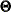. The population moments refer to the first-order moments (means) and the second-order central moments (variances of and covariances among the variables). Usually, the number of nonredundant population moments is greater than the number of model parameters for a structural model. The difference between the two is the degrees of freedom (df) of your model.

Formally, define a multiple-group situation where you have k independent groups in your model. The set of variables in each group might be different so that you have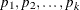manifest or observed variables for the k groups. It is assumed that the primary interest is to study the covariance structures. The inclusion of mean structures is optional for each of these groups. Define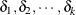as zero-one indicators of the mean structures for the groups. If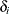takes the value of one, it means that the mean structures of group i is modeled. The total number of nonredundant elements in the moment matrices is thus computed by: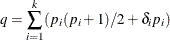The first term in the summation represents the number of lower triangular elements in the covariance or correlation matrix, while the second term represents the number of elements in the mean matrix. Let t be the total number of independent parameters in the model. The degrees of freedom is: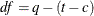where c represents the number of linear equality constraints imposed on the independent parameters in the model. In effect, the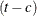expression means that each nonredundant linear equality constraint reduces one independent parameter.

#### Counting the Number of Independent Parameters

To count the number of independent parameters in the model, first you have to distinguish them from the dependent parameters. Dependent parameters are expressed as functions of other parameters in the SAS programming statements. That is, a parameter is dependent if it appears at the left-hand side of the equal sign in a SAS programming statement.

A parameter is independent if it is not dependent. An independent parameter can be specified in the main or subsidiary model specification statements or the PARAMETERS statement, or it is generated automatically by PROC CALIS as additional parameters. Quite intuitively, all independent parameter specified in the main or subsidiary model specification statements are independent parameters in the model. All automatic parameters added by PROC CALIS are also independent parameters in the model.

Intentionally or not, some independent parameters specified in the PARMS statement might not be counted as independent parameters in the model. Independent parameters in the PARMS statement belong in the model only when they are used to define at least one dependent parameter specified in the main or subsidiary model specification statements. This restriction eliminates the counting of superfluous independent parameters which have no bearing of model specification.

Note that when counting the number of independent parameters, you are counting the number of distinct independent parameter names but not the number of distinct parameter locations for independent parameters. For example, consider the following statement for defining the error variances in a LINEQS model:

variance    E1-E3 = vare1 vare2 vare3;


You define three variance parameter locations with three independent parameters vare1, vare2, and vare3. However, in the following specification:

variance    E1-E3 = vare vare vare;


you still have three variance parameter locations to define, but the number of independent parameter is only one, which is the parameter named vare.

#### Counting the Number of Linear Equality Constraints

The linear equality constraints refer to those specified in the BOUNDS or LINCON statement. For example, consider the following specification:

bounds  3 <= parm01 <= 3;
lincon  3 * parm02 + 2 * parm03 = 12;


In the BOUNDS statement, parm01 is constrained to a fixed number 3, and in the LINCON statement, parm02 and parm03 are constrained linearly. In effect, these two statements reduce two independent parameters from the model. In the degrees of freedom formula, the value of c is 2 for this example.

#### Adjustment of Degrees of Freedom

In some cases, computing degrees of freedom for model fit is not so straightforward. Two important cases are considered in the following.

The first case is when you set linear inequality or boundary constraints in your model, and these inequality or boundary constraints become active in your final solution. For example, you might have set inequality boundary and linear constraints as:

bounds  0 <= var01;
lincon  3 * beta1 + 2 * beta2 >= 7;


The optimal solution occurs at the boundary point so that you observe in the final solution the following two equalities:

   var01 = 0,
3 * beta1 + 2 * beta2 = 7


These two active constraints reduce the number of independent parameters of your original model. As a result, PROC CALIS will automatically increase the degrees of freedom by the number of active linear constraints. Adjusting degrees of freedom not only affects the significance of the model fit chi-square statistic, but it also affects the computation of many fit statistics and indices. See Dijkstra (1992) for a discussion of the validity of statistical inferences with active boundary constraints.

Automatically adjusting df in such a situation might not be totally justified in all cases. Statistical estimation is subject to sampling fluctuation. Active constraints might not occur when fitting the same model in new samples. If the researcher believes that those linear inequality and boundary constraints have a small chance of becoming active in repeated sampling, it might be more suitable to turn off the automatic adjustment by using the NOADJDF option in the PROC CALIS statement.

Another case where you need to pay attention to the computation of degrees of freedom is when you fit correlation models. The degrees-of-freedom calculation in PROC CALIS applies mainly to models with covariance structures with or without mean structures. When you model correlation structures, the degrees of freedom calculation in PROC CALIS is a straightforward generalization of the covariance structures. It does not take the fixed ones at the diagonal elements of the sample correlation matrix into account. Some might argue that with correlation structures, the degrees of freedom should be reduced by the total number of diagonal elements in the correlation matrices in the model. While PROC CALIS does not do this automatically, you can use the DFREDUCE=i option to specify the adjustment, where i can be any positive or negative integer. The df value is reduced by the DFREDUCE= value.

#### A Different Type of Degrees of Freedom

The degrees of freedom for model fitting has to be distinguished from another type of degrees of freedom. In a regression problem, the number of degrees of freedom for the error variance estimate is the number of observations in the data set minus the number of parameters. The NOBS=, DFR= (RDF=), and DFE= (EDF=) options refer to degrees of freedom in this sense. However, these values are not related to the degrees of freedom for the model fit statistic. The NOBS=, DFR=, and DFE= options should be used in PROC CALIS to specify the effective number of observations in the input data set only.